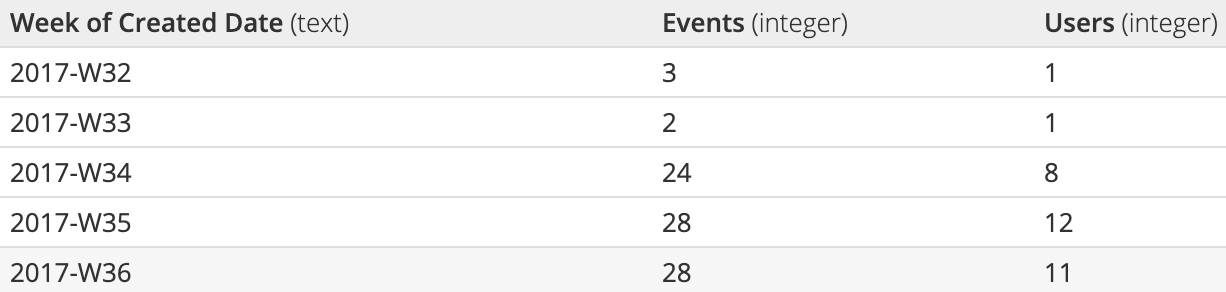# Table formats for Bar, Line, and Area charts

Bar, Area, and Line charts all accept a similar table format. Here’s a brief explanation of the types of table formats these charts accept, along with chart examples.

## In the Visual SQL interface

### One aggregated column and one grouped column

One aggregated column and one grouped column:

• The first column (the grouped column) maps to the x-axis
• The second column (the aggregated column) maps to the y-axis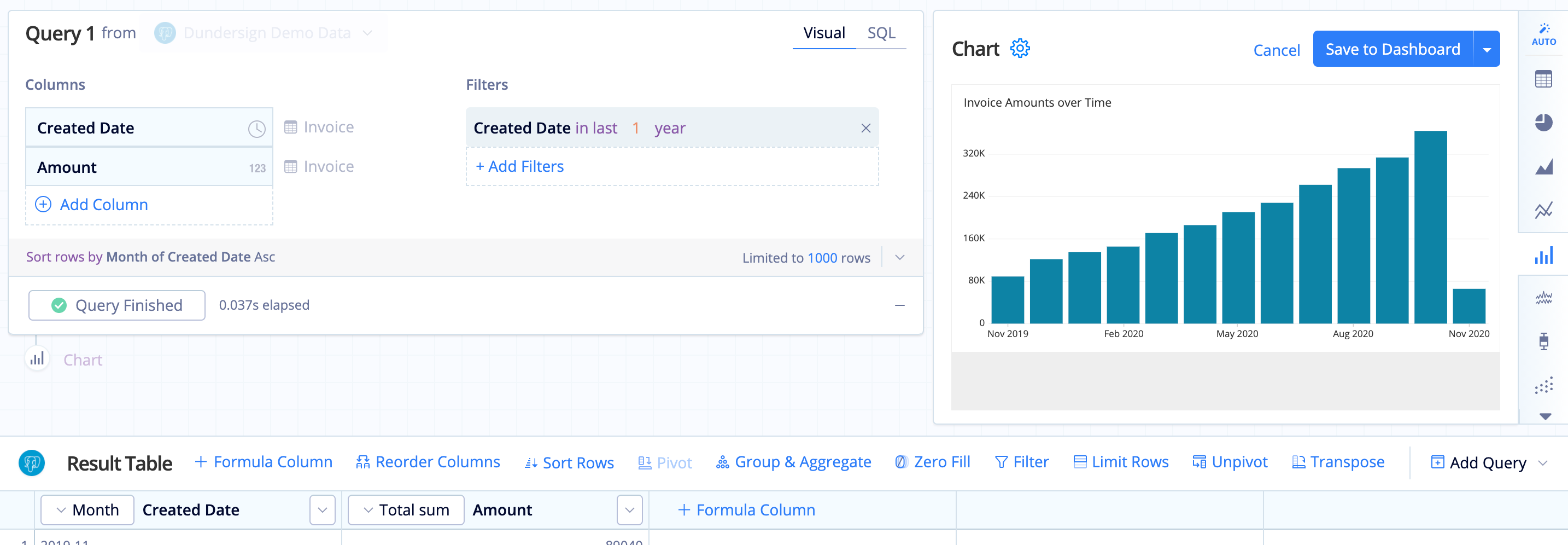### One aggregated column and two grouped columns

To make this result set compatible with Bar charts, you need to use a Pivot Action on the second column (the second grouped column). This turns the second column’s values into column headers.

• The first (grouped) column maps to the x-axis
• After the Pivot, subsequent columns map to the y-axis and are grouped and labeled by their column name.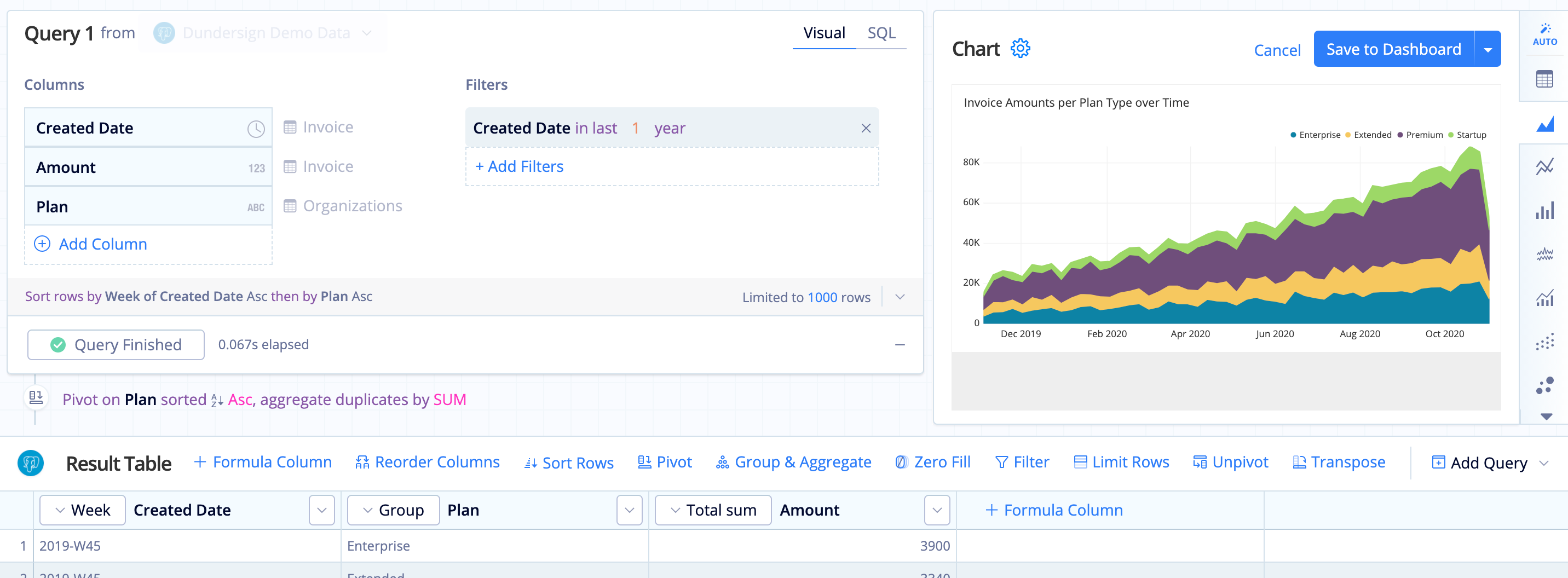### Multiple aggregated columns and one grouped column

• The first column maps to the x-axis
• Subsequent columns map to the y-axis and are grouped and labeled by their column name
• Tooltip displays second through last column’s values when hovering on the chart preview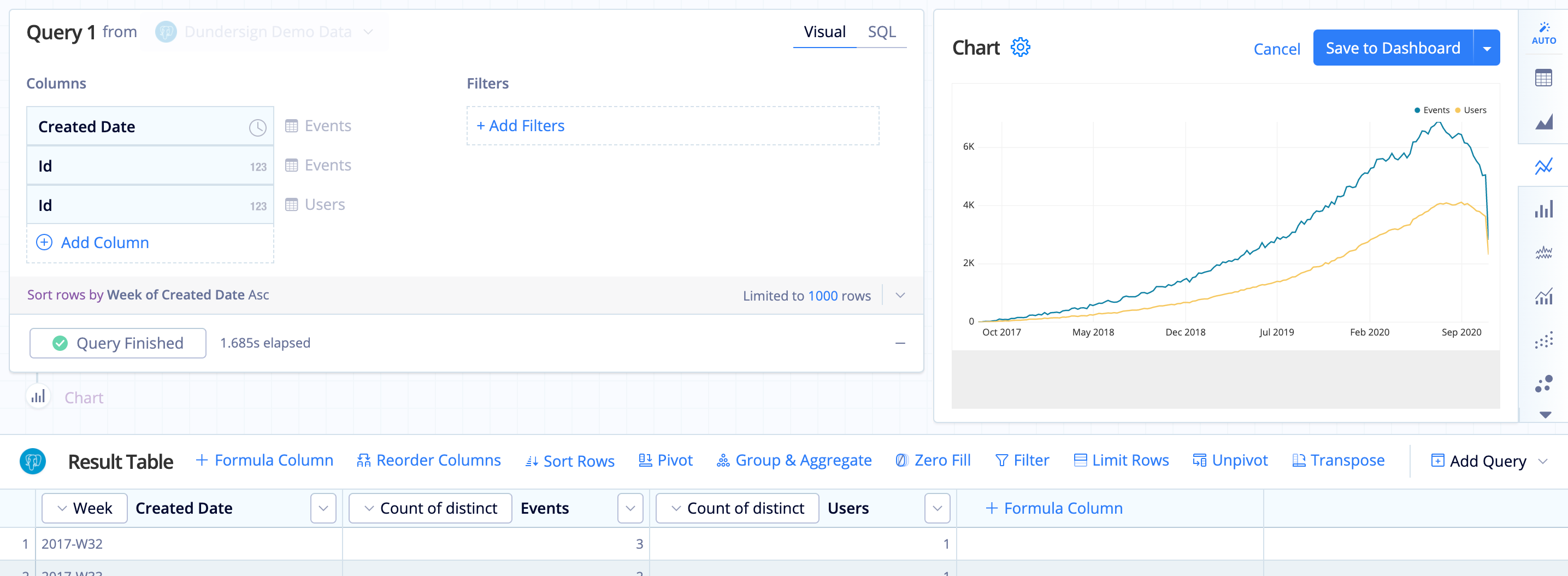### Number of lines in a Bar Line chart

For an example of Bar Line chart with multiple lines, check out our Dual axis Line charts article.

## In the Data Explorer interface

### One measure grouped by one dimension

• The first column maps to x-axis.
• The second column maps to y-axis.#### Sample table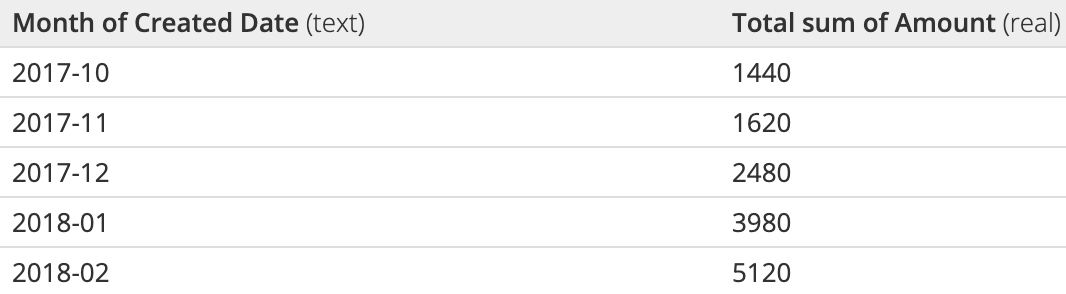### One measure grouped by two dimensions

• Add a Pivot step, which allows the second column’s values to each become a new column. View an example of a Pivot here.
• The first column maps to x-axis.
• After the Pivot, subsequent columns map to the y-axis and are grouped and labeled by their column name.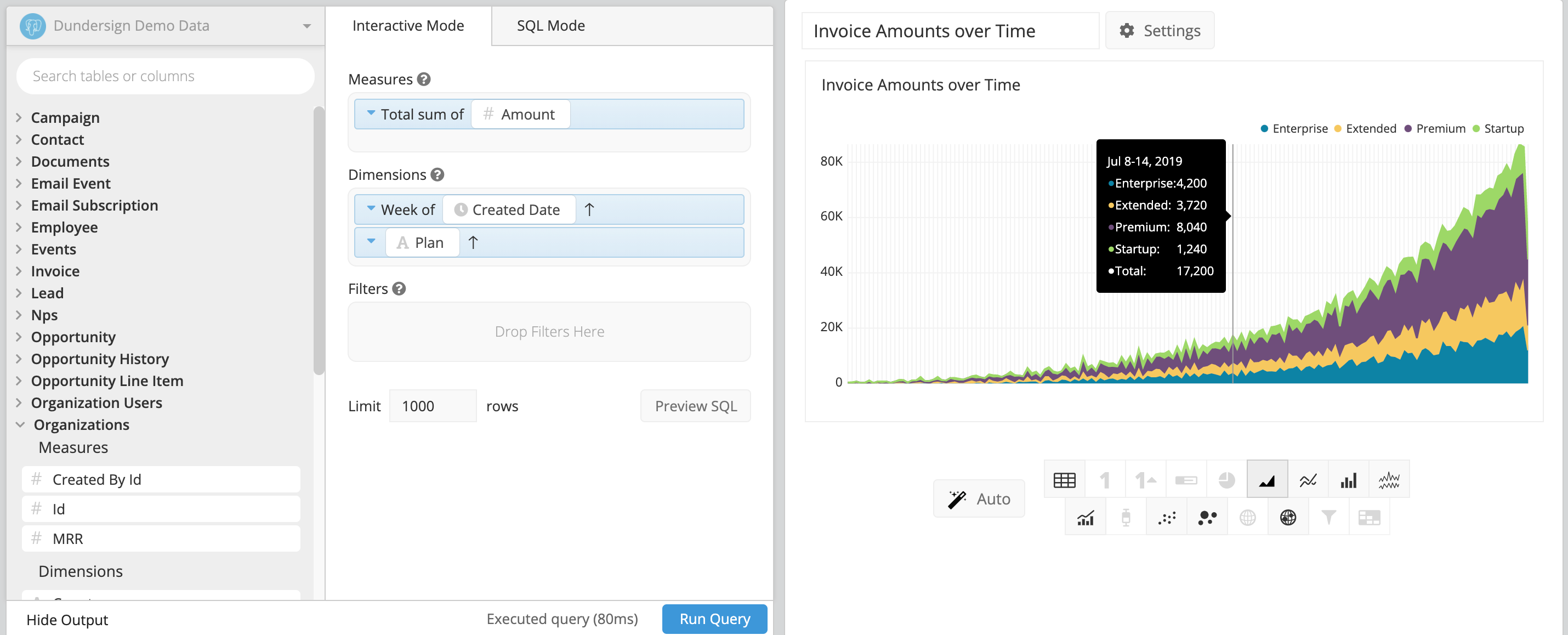#### Sample table (before and after Pivot step)### Pivot with two measures

First, let’s take a look at an example chart before and after a Pivot. Our example chart is grouped by two dimensions: Date and Customer. There are two aggregate columns: Amount and Count of Orders.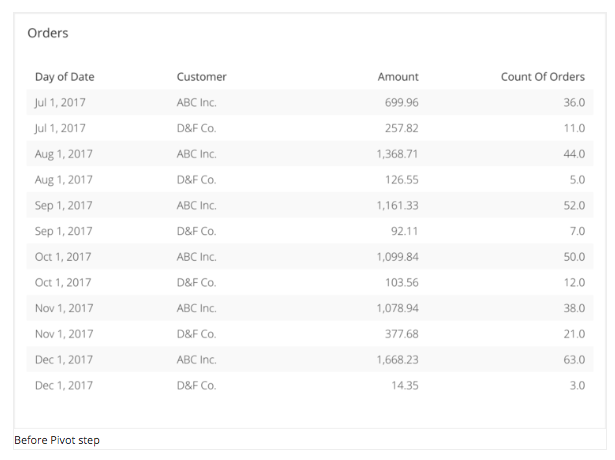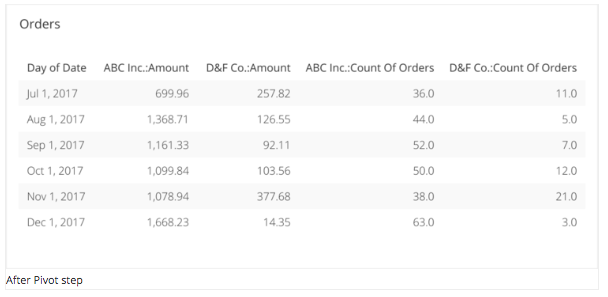Here’s what our chart looks like in the Data Explorer: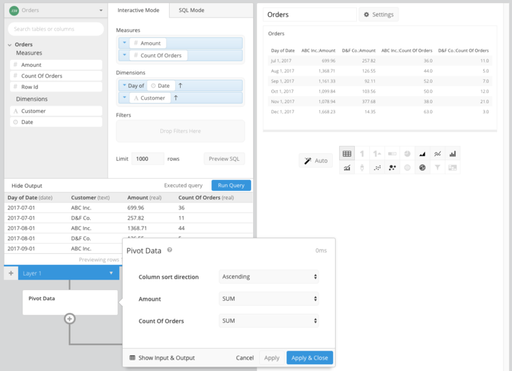The second dimension, Customer, is pivoted so the values are displayed/grouped with our column headers for our measures (Amount and Count of Orders). This type of pivot requires the following criteria:

• Grouped by exactly two dimensions
• Two or more measures (aggregates)
• Second (pivoted) dimension must have a limited number of values, otherwise, it will produce a large number of columns. (The limit in Chartio is 200 columns per table chart, though you would likely want far fewer than that to produce for legibility.)

### Multiple measures grouped by one dimension

• First column maps to x-axis.
• Subsequent columns are mapped to the y-axis, and are grouped and labeled by their column name.
• Tooltip displays second through last column on hover.#### Sample table